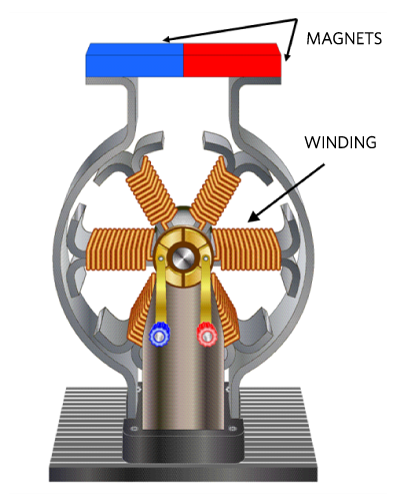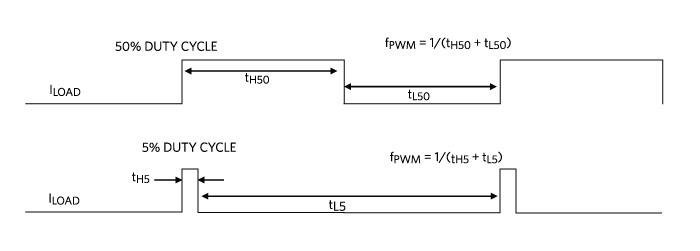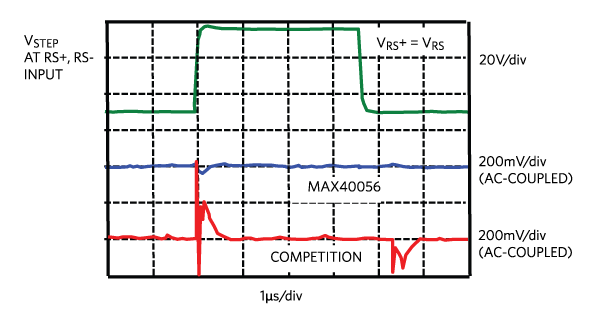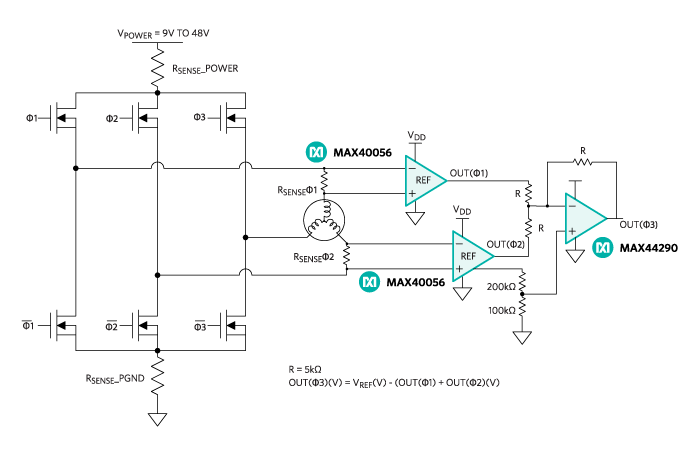# How Less Can Be More in Motor Control Design

### Abstract

The automobile is knee-deep in low-voltage DC motors throughout the vehicle’s framework. When creating an automotive BLDC motor system, the primary control challenge is to measure motor’s position and torque. This design solution defines DC motor signal dynamics and a new effective high PWM rejection current-sense amplifier (CSA) that has fast settling time accuracy to complement faster motor control algorithms.

#### Introduction

DC motors abound in industrial and automotive products. The automobile is knee-deep in low-voltage DC motors throughout the vehicle’s framework. For example, DC-motors provide adaptive headlights, power steering, cruise control, sunroof, wiper, and mirror adjustment functions (Figure 1).Figure 1. Numerous motors abound in automobiles including adaptive headlights.

The DC motor creates a moving magnetic field that uses a phase-driven architecture, that in turn produces the torque. When creating an automotive DC motor system, the primary control challenge is to measure position and torque.

One can capture these events by independently measuring each of the three inductive winding currents with individual current-sensing amplifiers (CSAs). This three-winding sensing solution requires independent CSAs that produce fast settling time and equally reject pulse-width-modulation (PWM) signals. Additionally, a microcontroller’s algorithms calculate instantaneous winding currents, while taking the PWM pulse phases or duty cycles into account.

It is exceedingly difficult to gain telematic information about the DC motor. This design solution defines DC motor signal dynamics to include PWM signal fast slew-rates, measurement settling times, and a new effective CSA that increases accuracy with fast settling times and high PWM rejection by simplifying the overall system.

#### PWM Signal Characteristics

The movement of electronic DC motors, such as a precise stepper motor, requires a PWM voltage or converted current signal to the motor’s windings. This voltage signal creates a magnetic field which repels or attracts the magnetic motor stator that causes motor rotations to occur (Figure 2).Figure 2. A six-pole DC motor uses positive (blue) and negative (red) magnets to repel or attract the winding’s PWM current instigated magnetic fields.

In Figure 2, a PWM voltage signal energizes the windings to create a magnetic field. This magnetic field interacts with the magnets to create the motor’s circular reaction.

The implementation of the PWM voltage signal (Figure 3) controls the motor speed with different frequencies and duty cycles.Figure 3. PWM voltage signal (ILOAD) with a 50% and 5% duty cycle.

In Figure 3, the frequency is equal to one divided by the summation of the signal’s high and low times. In high-precision motor applications, it is critical to determine motor speed. A 5% duty cycle averages a smaller magnetic field and hence a slower motor speed.

#### Sensing the Motor PWM Currents (ILOAD)

An expeditious way to sense the PWM signal is to place a small-value inline PCB resistor (RSENSE) and use a CSA to sense the voltage drop across this small resistor (Figure 4).Figure 4. CSA with a Kelvin-sensing PCB resistor.

Figure 4 shows a typical routing of Kelvin-sensed traces to the inputs of a CSA and then to a successive-approximation-register analog-to-digital converter or SAR-ADC.

The Kelvin-sense traces should be as close as possible to the current-sense resistor's solder contact pads. The range of RSENSE is dependent on the maximum magnitude of ILOAD, the CSA’s voltage gain (gain), and the CSA’s output-voltage range. The critical CSA performance specification is the amplifier settling time, and the critical SAR-ADC performance specification is the amount of time required to attain the output signal of the CSA or acquisition time.

#### Motor Control System

The proper configuration for this motor control system is to have the pairs of RSENSE and CSAs in all three current-sensing lines (Figure 5).Figure 5. Current sensing in a 3-phase servo motor.

In Figure 5, three pairs of power FETs, driven by the microcontroller, generate the load currents (ILOAD) to the motor windings. All three CSA devices connect to an ADC. These converters send their conversion data to a microcontroller to ascertain the phase and magnitude and then complete the feedback loop back to the power FETs.

#### PWM Common-Mode Voltages

High-speed motor applications require fast rising and falling PWM voltage signals. Figure 6 shows the output waveforms from two different CSAs when presented with a common-mode input delta 50V step.Figure 6. Common-mode step rejection to PWM step input with step input rise/fall time at 500V/µs.

In Figure 6, the CSA’s common-mode input-voltage change is approximately 50V with a 500V/µs rise and fall time. The two bottom curves exhibit the output response of two different CSAs. The maximum perturbation in the blue data line is approximately 50mV. The disruption in the red data line is approximately 600mV.

#### High-Speed, Low-Cost Motor Control System

The critical juncture in this system is the settling time of the CSA, acquisition speed of the SAR-ADC, and the cost of three CSA systems. Figure 7 shows a circuit that resolves these issues.Figure 7. Economic current sensing in a 3-phase servo motor by sensing two legs.

Summing the outputs of two CSAs, as shown in Figure 7, generates a voltage that represents the third winding's current. Per Kirchhoff’s Law, the third winding current equals the sum of the other two winding currents. A simple op-amp summing circuit is sufficient to produce a voltage that is proportional to the third winding current.

This circuit provides instantaneous winding currents of all three phases without further computation or knowledge of the PWM pulse phases or duty cycles and reduces the number of circuit CSAs from three to two.

#### Conclusion

The automotive industry’s prevalent use of low-voltage DC motors presents a difficult measurement challenge. An economical approach to finding the position and torque of each motor requires a new solution to this age-old problem. The MAX40056F uses an improved design technique to quickly provide an output signal to the controller. The lower settling time provides results with high-speed motor systems and makes a third CSA unnecessary. This new design architecture provides excellent performance without breaking the bank.

MAX40056EVKIT
MAX44290EVKIT

####View Video

DC Motor Current Sensing in a PWM Environment with the MAX40056 Current-Sense Amplifier